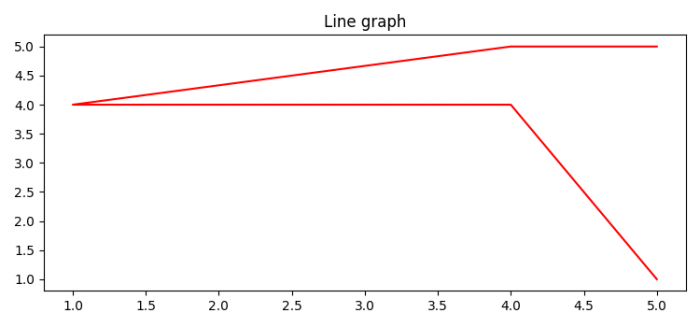# How to plot an array in Python using Matplotlib?

To plot an array in Python, we can take the following steps −

• Set the figure size and adjust the padding between and around the subplots.
• Create two arrays, x and y, using numpy.
• Set the title of the curve using title() method.
• Plot x and y data points, with red color.
• To display the figure, use show() method.

## Example

import numpy as np
import matplotlib.pyplot as plt

plt.rcParams["figure.figsize"] = [7.50, 3.50]
plt.rcParams["figure.autolayout"] = True

x = np.array([5, 4, 1, 4, 5])
y = np.sort(x)

plt.title("Line graph")
plt.plot(x, y, color="red")

plt.show()

## Output